# 16 Best Discrete Mathematics books That You Must Read

Discrete mathematics means mathematical structures are discrete rather than continuous. It is a branch of mathematics dealing with objects which do not vary smoothly in this way but have distinct, separated value.

Here you will get some of the best discrete mathematics books.

## 1. Discrete Mathematics and Its Applications

This world-renowned best-selling text was written to accommodate the needs across a variety of majors and departments, including mathematics, computer science, and engineering. As the market leader, the book is highly flexible, comprehensive and a proven pedagogical teaching tool for instructors. Digital is becoming increasingly important and gaining popularity, crowning Connect as the digital leader for this discipline.

McGraw-Hill Education's Connect, available as an optional add on item, is the only integrated learning system that empowers students by continuously adapting to deliver precisely what they need, when they need it and how they need it - ensuring class time is more effective. Connect allows the professor to assign homework, quizzes, and tests easily and automatically grades and records the scores of the student's work. Problems are randomized to prevent sharing of answers and may also have a "multi-step solution" which helps move the students' learning along if they experience difficulty.

## 2. Discrete Mathematics with Applications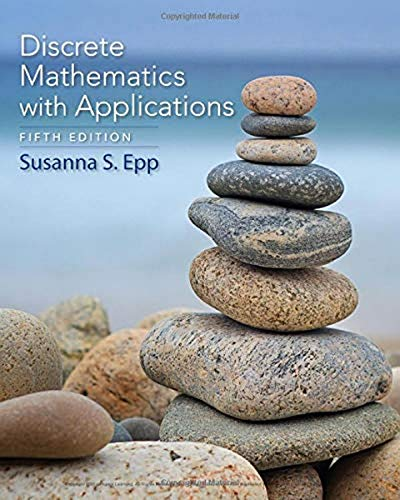Author: Epp, Susanna S.
Published at: 01/01/2019
ISBN: 1337694193

DISCRETE MATHEMATICS WITH APPLICATIONS, 5th Edition, explains complex, abstract concepts with clarity and precision and provides a strong foundation for computer science and upper-level mathematics courses of the computer age. Author Susanna Epp presents not only the major themes of discrete mathematics but also the reasoning that underlies mathematical thought. Students develop the ability to think abstractly as they study the ideas of logic and proof.

While learning about such concepts as logic circuits and computer addition, algorithm analysis, recursive thinking, computability, automata, cryptography and combinatorics, students discover that the ideas of discrete mathematics underlie and are essential to today's science and technology.

## 3. Practical Discrete Mathematics: Discover math principles that fuel algorithms for computer science and machine learning with Python

A practical guide simplifying discrete math for curious minds and demonstrating its application in solving problems related to software development, computer algorithms, and data science

#### Key Features

• Apply the math of countable objects to practical problems in computer science
• Explore modern Python libraries such as scikit-learn, NumPy, and SciPy for performing mathematics
• Learn complex statistical and mathematical concepts with the help of hands-on examples and expert guidance

## 4. Proofs: A Long-Form Mathematics Textbook (The Long-Form Math Textbook Series)

This textbook is designed for students. Rather than the typical definition-theorem-proof-repeat style, this text includes much more commentary, motivation and explanation. The proofs are not terse and aim for understanding the economy. Furthermore, dozens of proofs are preceded by "scratch work" or a proof sketch to give students a big-picture view and an explanation of how they would come up with it on their own. This book covers intuitive proofs, direct proofs, sets, induction, logic, the contrapositive, contradiction, functions and relations. The text aims to make the ideas visible and contains over 200 illustrations. The writing is relaxed and conversational and includes periodic attempts at humour. This text is also an introduction to higher mathematics. This is done in part through the chosen examples and theorems.

Furthermore, following every chapter is an introduction to an area of math. These include Ramsey theory, number theory, topology, sequences, real analysis, big data, game theory, cardinality and group theory. After every chapter is "pro-tips," which are short thoughts on things I wish I had known when I took my intro-to-proofs class. They include finer comments on the material, study tips, historical notes, comments on mathematical culture, and more. Also, after each chapter's exercises is an introduction to an unsolved problem in mathematics. In the first appendix we discuss some further proof methods, the second appendix is a collection of particularly beautiful proofs, and the third is some writing advice.

## 5. Concrete Mathematics: A Foundation for Computer Science (2nd Edition)

This book introduces the mathematics that supports advanced computer programming and the analysis of algorithms. The primary aim of its well-known authors is to provide a solid and relevant base of mathematical skills - the skills needed to solve complex problems, to evaluate horrendous sums, and to discover subtle patterns in data. It is an indispensable text and reference not only for computer scientists - the authors themselves rely heavily on it! - but for serious users of mathematics in virtually every discipline.

Concrete Mathematics is a blending of CONtinuous and disCRETE mathematics. "More concretely," the authors explain, "it is the controlled manipulation of mathematical formulas, using a collection of techniques for solving problems." The subject matter is primarily an expansion of the Mathematical Preliminaries section in Knuth's classic Art of Computer Programming, but the style of presentation is more leisurely, and individual topics are covered more deeply. Several new topics have been added, and the most significant ideas have been traced to their historical roots. The book includes more than 500 exercises, divided into six categories. Complete answers are provided for all exercises, except research problems, making the book particularly valuable for self-study.

## 6. Discrete Mathematics: An Open Introduction

This gentle introduction to discrete mathematics is written for first and second-year math majors, especially those who intend to teach. The text began as a set of lecture notes for the discrete mathematics course at the University of Northern Colorado. This course serves both as an introduction to topics in discrete math and as the "introduction to proof" course for math majors. The course is usually taught with a large amount of student inquiry, and this text is written to help facilitate this.

Four main topics are covered: counting, sequences, logic, and graph theory. Along the way, proofs are introduced, including proofs by contradiction, proofs by induction, and combinatorial proofs. The book contains over 470 exercises, including 275 with solutions and over 100 with hints. There are also Investigate! activities throughout the text to support active, inquiry-based learning.

## 7. Discrete Mathematics with Applications 4th Fourth Edition

Discrete Mathematics with Applications is a great book with examples, exercises and knowledge checks. In this book, Susanna S. Epp explains the topics thoroughly and rigorously in a straightforward manner.

This book highlights the really important parts of each chapter and contains a bunch of useless color photographs, charts, diagrams, tables, vignettes, and other "educational aid" fluff that is all the rage these days with publishers.

The key topics are

• The logic of compound statements
• The logic of quantified statements
• Elementary number theory and methods of proof
• Sequences and mathematical induction
• Set theory
• Counting
• Functions
• Recursion
• O-Notation and the efficiency of algorithms
• Relations
• Graphs and trees.

## 8. Discrete Mathematics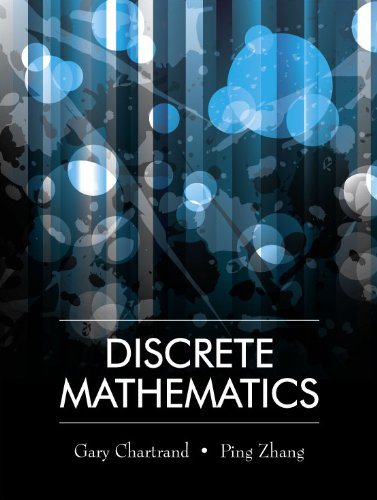Author: Gary Chartrand,Ping Zhang
Published at: 20/03/2011
ISBN: 1577667301

A student-friendly introduction to discrete mathematics that includes more than 1,600 exercises, ranging from elementary to challenging, is included with hints/answers to all odd-numbered exercises, accessible and lively descriptions of proof techniques.

Logic, relations and functions, algorithms and analysis of algorithms, introduction to number theory, combinatorics, discrete probability, partially ordered sets, lattices and Boolean algebras, cryptography, and finite-state machines are also included to help the students.

Important coverage are

• What Is discrete mathematics?
• Logic statements
• Sets
• Methods of proof
• Mathematical induction
• Relations and functions
• Algorithms and complexity
• Integers
• Introduction to counting
• Discrete probability
• Partially ordered sets and boolean algebra
• Introduction to graphs
• Trees
• Planar graphs and graph colorings.

## 9. Mathematics: A Discrete Introduction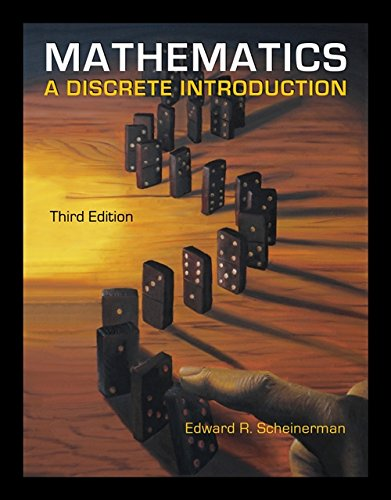Author: Edward A. Scheinerman
Published at: 05/03/2012
ISBN: 0840049420

This book contains self-tests, lots of hints and comments, some answers, solutions to self-tests that really helped you to understand the material better.

By reading this book you will learn the importance of discrete mathematics in the fields of computer science, engineering, probability, statistics, operations research, and other areas of applied mathematics.

Some coverages are

• Speaking of mathematics
• Boolean Algebra
• Lists
• Factorial
• Operations
• Combinatorial proof
• Relations
• Equivalence relations
• Partitions
• Binomial coefficient
• Counting multisets
• Inclusion-exclusion
• Smallest counterexample
• Recurrence relations
• The Pigeonhole principle.

There are many self-test that will make you confident on the exam day.

## 10. Introductory Discrete Mathematics (Dover Books on Computer Science)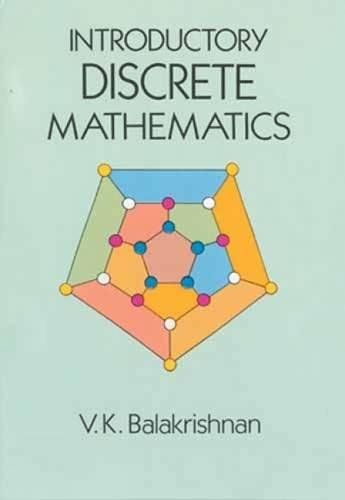Author: V. K . Balakrishnan
Published at: 18/10/2010
ISBN: 0486691152
This is the best book for undergraduate students in computer science and mathematics. This is an introductory book for students as the name suggests.

The theory of computational complexity and NP-completeness is outlined in the appendix. There are many algorithms to solve mathematical expressions.

This book covers the following important topics
• Fundamental operations involving sets and the principle of mathematical induction
• Basic counting principles
• Permutations
• Combinations
• The inclusion-exclusion principle
• Generating functions
• Recurrence relations
• Introduction to the analysis of algorithms
• Graph theory with applications to some stand network optimization problems
• Algorithms to solve these problems.

## 11. Discrete Mathematics (Quick Study Academic)

This is a reference tool for professors, teachers, and students throughout a course. It's a six-page guide used in business and also present in most middle & high school math competitions.

This must-have quick reference will be your success tool.

This guide includes

• Graphs & trees
• Functions & set relations
• Counting
• Sets
• Mathematical induction
• Sequences
• Methods of proof
• Proving universal statements
• Quantified statements
• Arguments
• The logic of statements.

## 12. Discrete Mathematics for Computer Science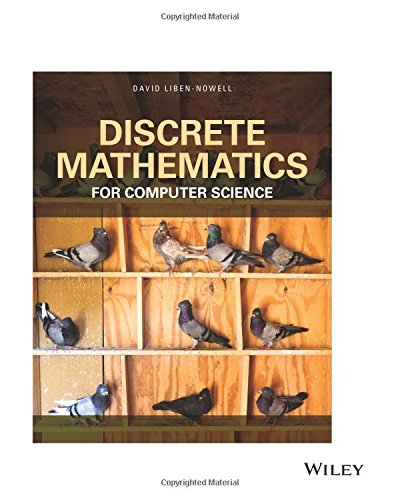Author: David Liben-Nowell
Published at: 20/07/2017
ISBN: 1119441854

This guide includes many important topics including basic data types, logic, proofs, mathematical induction, analysis of algorithms, number theory, relations, counting, probability, graphs, and trees.

It's a great book for computer science students.

Discrete Mathematics for Computer Science provides you

• A comprehensive treatment of standard course topics for the introductory discrete mathematics course
• The relationship between the concepts and their application to computer science
• A wide selection of exercises
• Detailed exploration problems
• Examples and problems inspired by wide-ranging applications of computer science
• Over 450 examples
• Chapter summaries
• Simple quizzes
• Approximately 1600 homework exercises of widely varying difficulty.

## 13. Discrete Mathematics (8th Edition)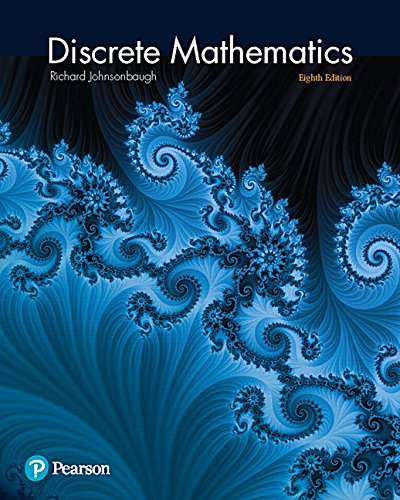Author: Richard Johnsonbaugh
Published at: 16/03/2017
ISBN: 0321964683
 Discrete Mathematics provides computer science applications in a clear, high-quality format. It also includes exercise sets including basic exercises, advanced exercises, and computer exercises, which are designed to allow your students to master what they have learned before moving on to more difficult material.Important coverage areSets and logicProofsFunctions, sequences, and relationsAlgorithmsIntroduction to number theoryCounting methods and the pigeonhole principleRecurrence relationsGraph theoryTreesNetwork modelsBoolean algebras and combinatorial circuitsAutomata, grammars, and languagesComputational geometry.

## 14. Discrete Mathematics and Applications (Textbooks in Mathematics)

Discrete Mathematics and Applications emphasizes proofs, which will appeal to a subset of this course market. It covers trees, algorithms and focuses on graph theory.

The supporting materials in this book will help you get an outstanding number on the exam. This guide is used for mathematics, mathematics education, and computer science majors. It consists of two parts.

Part one focuses on

• How to write proofs
• Then moves on to topics in number theory
• employing set theory in the process.

Part two focuses on

• Computations
• Combinatorics
• Graph theory
• Trees, and algorithms.

## 15. Mathematics: A Discrete Introduction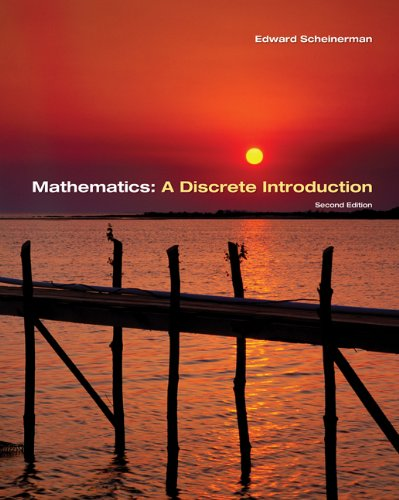Author: Edward A. Scheinerman
Published at: 06/06/2005
ISBN: 0534398987

This textbook is really a great intro from counting to basic cryptography to graph theory. After reading this book you will be able to understand both the topic and the construction of the book.

This book gives two primary objectives

• It teaches students fundamental concepts in discrete mathematics
• It teaches students proof-writing skills.

You'll know

• how to write proofs
• how to think clearly
• To present cases logically beyond this course.
• The usefulness of discrete mathematics is very useful.

## 16. Discrete Mathematics: An Open Introduction for Teachers

This guide is specially written for those who will go on to teach and for a first or second-year undergraduate course for math majors.

This guide covers

• 363 exercises
• 233 with answers or full solutions
• 130 more involved problems suitable for homework
• A full index and a list of symbols
• Examples of important definitions and theorems inboxes.

Covered Four main topics are

1. Counting
2. Sequences
3. Logic
4. graph theory.

Thanks for reading this post. If you have any opinion don't hesitate to comment here. Also please subscribe our newsletter to get more updates.